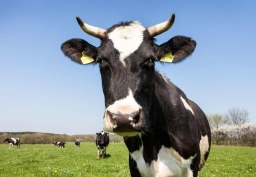There are a number of unspecified numbers of cows and hens in a den. If there are a total of 30 heads and 80 legs in the den, find the number of cows and hens in the den.

a =  10
b =  20

### Step-by-step explanation:

a+b=30
4a+2b=80

a+b=30
4·a+2·b=80

a+b = 30
4a+2b = 80

Pivot: Row 1 ↔ Row 2
4a+2b = 80
a+b = 30

Row 2 - 1/4 · Row 1 → Row 2
4a+2b = 80
0.5b = 10

b = 10/0.5 = 20
a = 80-2b/4 = 80-2 · 20/4 = 10

a = 10
b = 20

Our linear equations calculator calculates it.Did you find an error or inaccuracy? Feel free to write us. Thank you!

Tips for related online calculators
Do you have a system of equations and looking for calculator system of linear equations?